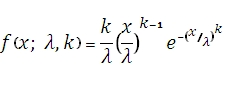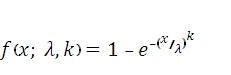# SQL Server WEIBULL function

Updated: 9 August 2010

Use WEIBULL to calculate the Weibull distribution. The formula for the probability density function (pdf) is:Where
x              is the value at which to evaluate the function
k              is the shape parameter
λ                  is the scale parameter
The formula for the cumulative distribution function (cdf) is:Syntax
SELECT [wctStatistics].[wct].[WEIBULL] (
<@X, float,>
,<@Alpha, float,>
,<@Beta, float,>
,<@Cumulative, bit,>)
Arguments
@X
is the value at which to evaluate the function. @X is an expression of type float or of a type that can be implicitly converted to float
@Alpha
is the shape parameter of the distribution. @Alpha is an expression of type float or of a type that can be implicitly converted to float
@Beta
is the scale parameter of the distribution. @Beta is an expression of type float or of a type that can be implicitly converted to float
@Cumulative
is a logical value that determines if the probability density function (False, 0) or the cumulative distribution function (True, 1) is being calculated.
Return Types
float
Remarks
·         If @X < 0, WEIBULL returns an error.
·         If @Alpha ≤ 0 or @Beta ≤ 0, WEIBULL returns an error.
·         WEIBULL(@X,1,@Beta,@Cumulative)= EXPONDIST(@X,1/@Beta, @Cumulative)
Examples

select
wct.WEIBULL(105, 20, 100, 'True')

This produces the following result

----------------------
0.929581390069277

(1 row(s) affected)

select
wct.WEIBULL(105, 20, 100, 'False')

This produces the following result

----------------------
0.0355888640245043

(1 row(s) affected)

select
wct.WEIBULL(6, 1, 10, 'False')

This produces the following result

----------------------
0.0548811636094027

(1 row(s) affected)

select
wct.EXPONDIST(6, 1.0000/10, 'False')

This produces the following result

----------------------
0.0548811636094026

(1 row(s) affected)

### SupportCopyright 2008-2023 Westclintech LLC         Privacy Policy        Terms of Service